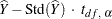### Confidence Limits for the Total

If you specify the keyword CLSUM, the procedure computes confidence limits for the total. The confidence coefficient is determined by the value of the ALPHA= option, which by default equals 0.05 and produces 95% confidence limits. The confidence limits are computed as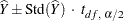where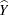is the estimate of the total,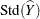is the estimated standard deviation, and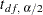is the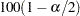th percentile of the t distribution with df calculated as described in the section t Test for the Mean.

If you specify the keyword UCLSUM, the procedure computes the one-sided upper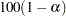% confidence limit for the sum: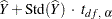If you specify the keyword LCLSUM, the procedure computes the one-sided lower% confidence limit for the sum: